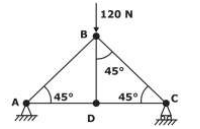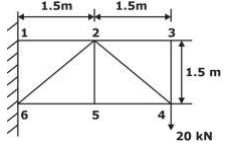Trusses homework Help at TutorEye

## Top Questions

assuming a contact area of 1 cm^2 (1 x 10^-4 m^2). Recall P = F/A and 1 Pa = 1 N/m^2. a) In Pascals (Pa) and b) In kilopascals (kPa)
View More

down from where you want to be. That means your GPS has to be precise. Really precise. It relies on atomic clocks on-board the satellites. These clocks are precise to the nanosecond scale. Your phone accessing the GPS signal is the Earth based observer. Do you expect the atomic clock to run slower or faster than the clock on your phone? Is the time on the atomic clock the proper time or dilated time? Is the time on your phone the proper time or dilated time?
View More

r if my data won’t work and I need to set up a new experiment
View More

r practice: friction and energy worksheet
View More

## Trusses:

Trusses are rigid structures composed of several straight members pin joined to each other. These are a series of triangular structures lying on the same plane. It can sustain static and dynamic load, without any relative motion or strength failure. It contains one end hinged and other end roller supported. Trusses are foundational members for building bridges, roofs and towers.

## TrussesSample Questions:

Question 1: A three dimensional arrangement of members to withstand high load at a very long span is

a) Plane truss              b) Rigid truss

c) Space truss             d) Cantilever truss

Explanation: Space trusses are three

Question 2: Which of the following is true in the context of solving truss by method of section?

a) A section can cut any number of members with maximum 3 unknown forces in members.

b) A section can cut any number of members with a maximum 4 unknown forces in members.

c) A section can cut any number of members with a maximum of 5 unknown forces in members.

d) All of the above

Answer: a) A section can cut any number of members with maximum 3 unknown forces in members.

Explanation: Solving a truss problem by method of section, a section can cut any member

Question 3: For cantilever truss, governing equation is

a) m = 2j + 4               b) m = 2j - 1

c) m = 2j + 1               d) m = 2j - 4

Answer: d) m = 2j - 4

Explanation: The cantilever truss is governed by the equation m = 2j - 4

Question 4: The governing equation for rigid or collapsible truss is

a) m < 2j - 3             b) m < 2j - 2

c) m < 2j - 1             d) m < 2j - 4

Answer: a) m < 2j - 3

Explanation: Rigid or collapsible truss is governed

Question 5: Which of the following assumptions made in the truss analysis is false?

a) The truss is loaded and supported only at its joints.            b) Truss can be considered a coplanar force system.

c) The forces in the members of the truss are purely axial.    d) None of these

Explanation: Trusses are only loaded and pin supported at its joints.

Question 6: A truss is subjected to a vertical force of 120 N at joint B as shown in the figure. The length of each member is 1m. The magnitude of force in the member BD isa) 20 N           b) 10 N

c) 0 N             d) 12 N

Explanation:  The members AD and BD are Collinear, therefore the force

Question 7: For the pin joint truss, find the axial force in the member 2-5.a) zero                  b) one

c) infinite             d) none of these

Explanation: If at a joint, three members or three forces act

Question 8: Truss problems can be solved using _________.

a) Method of joints                b) Method of sections

c) Both (a) and (b)                d) None of these

Answer: c) Both (a) and (b)

Explanation: Truss problems are solved using two methods

Question 9: The governing equation of perfect truss is

a) m = 2j - 3              c) m > 2j - 3

b) m < 2j - 3              d) m > 3j - 2

Answer: a) m = 2j - 3

Explanation: The governing equation of perfect truss, m = 2j - 3

Question 10: The method of _________ is a technique in which the force equilibrium is checked at respectable joints of the truss system.

a) section                            b) joints

c) both (a) and (b)               d) none of these

Explanation: In the method of joints, the